# Test: Electromagnetic Waves- 2

## 20 Questions MCQ Test Topicwise Question Bank for Electrical Engineering | Test: Electromagnetic Waves- 2

Description
Attempt Test: Electromagnetic Waves- 2 | 20 questions in 60 minutes | Mock test for Electrical Engineering (EE) preparation | Free important questions MCQ to study Topicwise Question Bank for Electrical Engineering for Electrical Engineering (EE) Exam | Download free PDF with solutions
QUESTION: 1

### The propagation constant γ for a material with μr = 1, εr = 8, σ = 0.25 pS/m and having the wave frequency of 1.6 MHz would be approximately equal to

Solution:

Given,
σ = 0.25 pS/m; μr = 1; εr = 8;
f = 1.6 x 106 Hz
Now,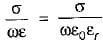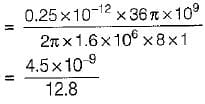or,   σ/ωε = 0.3515 x 10-9 ≈ 0
or, σ  ≈ 0
Since σ = 0, therefore the material will behave as a perfect dielectric (α = 0).
∴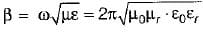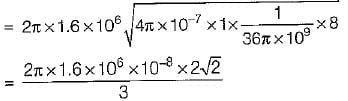∴ Propagation constant,
γ = α + jβ = 0 + j 9.471 x 10-2
≈ j.095 m-1

QUESTION: 2

### What is the skin depth in copper at 10 GHz? Assuming conductivity of copper σ = 5.8 x 107 mhos/m and permeability equal to that of free space.

Solution:

Skin depth,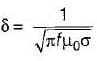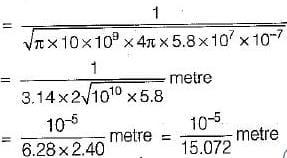= 0.66 x 10-6 m = 0.66μm

QUESTION: 3

### The velocity of a plane wave in a lossless medium having a relative permittivity of 4 and relative permeability of unity would be

Solution:

For a lossless or perfect medium, velocity of plane wave is given by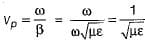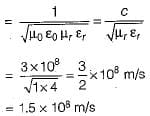QUESTION: 4

An elliptically polarized wave travelling in the +z direction in air has x and y components
Ex = 2 sin(ωt - βz) V/m;
Ey = 4 sin(ωt - βz + 75°) v/m
The average power per unit area conveyed by the wave is

Solution:

The average power per unit area is equal to the Poynting vector, the magnitude of which is given by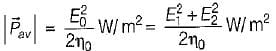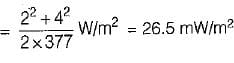QUESTION: 5

Consider the following statements:
1. A wave whose phase is constant over a set of planes is called plane wave.
2. A wave whose phase is constant while magnitude is variable is called uniform plane wave.
3. The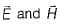fields are non-uniform over plane surfaces.
4. Earth is considered as good conductor in one part of the radio frequency range and as dielectric in other frequency range.
Which of the above statements is/are not correct?

Solution:
• A wave whose phase and magnitude both are constant is called uniform plane wave. Hence, statement-2 is not correct.
•  A uniform plane is one in whichlie in a plane and have the same value everywhere in that plane at any fixed instant.
• Thefields are uniform over plane surfaces. Hence, statement-3 is also not correct.
QUESTION: 6

Assertion (A): Displacement current directly passes through the capacitor.
Reason (R): The current through a capacitive element is called displacement current.

Solution:

Assertion is not correct because it is not the displacement current which directly passes through a capacitor but it is only apparant current representing the rate at which the flow of charge takes place from one plate of the capacitor to the other plate.

QUESTION: 7

In a homogeneous, isotropic, linear and stationary media, a plane electromagnetic wave has the following characteristics.

Solution:

In a homogeneous, isotropic, linear and stationary media, ∇·E = ρ = 0 and both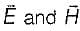are transverse.

QUESTION: 8

Assertion (A): The distance to which a wave penetrates a conductor is known as depth of penetration.
Reason (R): The depth of penetration (δ) is defined as that depth in which the wave has been attenuated to ‘e’ percent of its original value.

Solution:

The depth of penetration (δ) is defined as that depth in which the wave has been attenuated to ‘1/e’ percent (37%) of its original value. Hence, reason is not a correct statement.

QUESTION: 9

The polarization of a dielectric is given by

Solution:
QUESTION: 10

In a travelling electrom agnetic wave, E and H vector fields are

Solution:
QUESTION: 11

A wave is incident normally on a good conductor. If the frequency of a plane electromagnetic wave increases four times, the skin depth, will

Solution:

Skin depth (δ)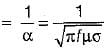∴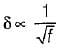When the frequency is increased 4-times, then skin depth will become half.

QUESTION: 12

The electric field of a travelling wave is given by 100 cos(109t - 4x). The velocity and the wavelength are respectively.

Solution:

Given,
E = 100 cos (109t - 4x)
or, E = E0 cos (ωt - βx)
and β = 4
Thus, ve lo city of propag ation is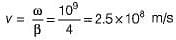and wavelength,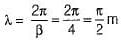QUESTION: 13

In a dielectric-conductor boundary (interface), the tangential component of electric field is

Solution:
QUESTION: 14

Which of the following statements is not true of waves in general?

Solution:

A wave can’t be a function of time only. It is function of both space and time.

QUESTION: 15

When an EM wave is incident on a dielectric, it is

Solution:
QUESTION: 16

Poynting vector gives

Solution:

The quantity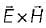is known as Poynting vector, measured in watt/m2.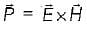Poynting vector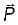represents the instantaneous power density vector associated with the EM field at a given point, in other words, it gives rate of energy flow.

QUESTION: 17

Match List-I with List-II and select the correct answer using the codes given below the lists:
List-I
A. Free space
B. Lossless dielectrics
C. Lossy dielectrics
D. Good conductors
List-lI
1. σ ≠ 0, ε = ε0εr, μ = μ0μr
2. σ = 0, ε = ε0, μ = μ0
3. σ ≈ ∞, ε = ε0, μ = μ0μr
4. σ = 0, ε = ε0εr, μ = μ0μr

Codes:
A B C D
(a) 4 2 3 1
(b) 2 4 1 3
(c) 4 2 1 3
(d) 2 4 3 1

Solution:
QUESTION: 18

Match List-I (EM phenomena) with List-ll (Uses) and select the correct answer using the codes given below the lists:
List - I
A. Cosmic rays
D. Gamma rays
List - II
1. Sterilization
2. Cancer therapy
3. Astronomy
4. Photography
Codes:
A B C D
(a) 4 3  2 1
(b) 3 4  1 2
(c) 1 2  3 4
(d) 3 1 4  2

Solution:
QUESTION: 19

A lossy dielectric has an intrinsic impedance of 200∠30° Ω at a particular frequency. If, at that frequency, the plane wave propagating through the dielectric has the magnetic field component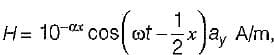the skin depth will be

Solution:

Given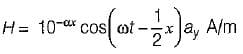Here,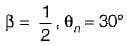Now,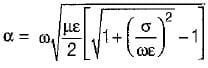and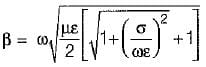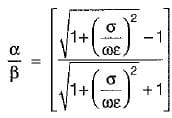But,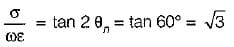Hence,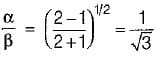or,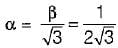So, skin depth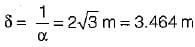QUESTION: 20

What is the major factor for determining whether a mediam is free space, lossless dielectric, or good conductor?

Solution:Use Code STAYHOME200 and get INR 200 additional OFF Use Coupon Code